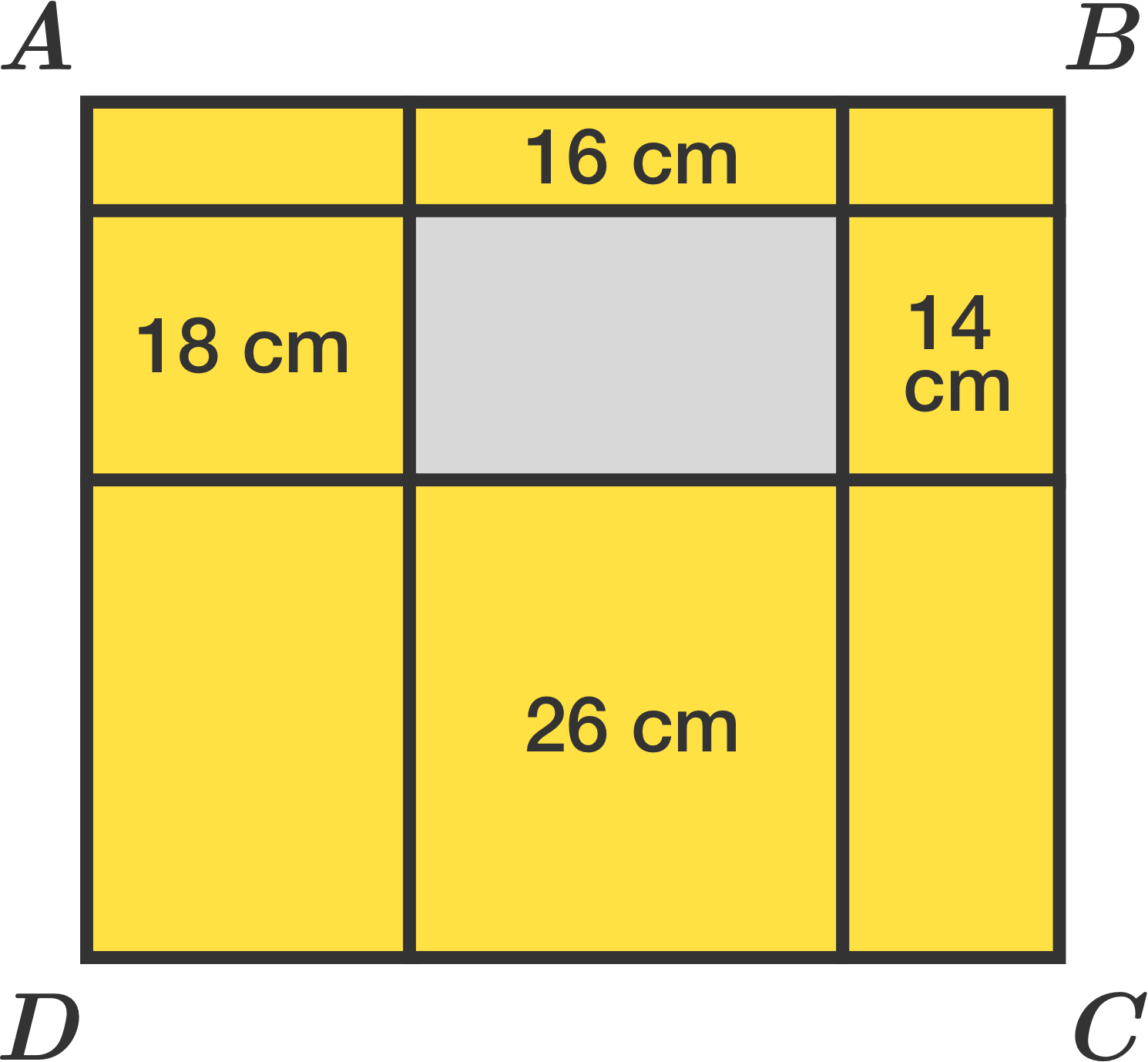# Perimeters Provided Around In The Vicinity Of Its Perimeter

Geometry Level 1The rectangle $ABCD$ was divided into 9 small rectangles, and some rectangles have their perimeter inside it. The perimeter of rectangle $ABCD$ is $54 \text{ cm}$. What is the perimeter of the gray rectangle?

×